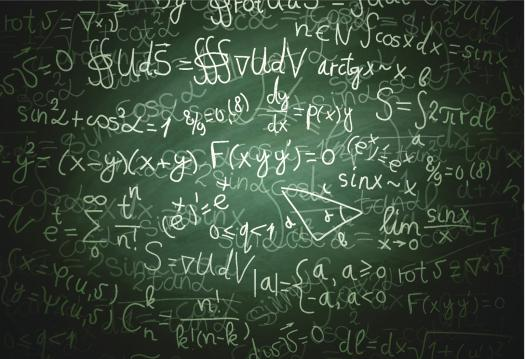# How Good Are You In Measures Of Central Tendency Math?

10 Questions | Total Attempts: 33SettingsAlso known as measures of central location, a measure of central tendency is a single value that attempts to describe a set of data by identifying the central position within that set of data. Well known measures of central tendency are the Mean, the Median, and Mode. Do you think you master the rules of this portion of Mathematics? If yes, try our quiz and find out.

• 1.
How do you define the Mean?
• A.

It is equal to the sum of all the values in the data set divided by the number of values in the data set.

• B.

It is the average value of a data set.

• C.

It is the highest values of a data set.

• D.

It is the lowest value of a data set.

• 2.
How would you define the Median?
• A.

It is the middle point for a set of data that has been arranged in order of magnitude

• B.

It is the highest score for a set of data that has been arranged in order of magnitude

• C.

It is the middle score for a set of data that has been arranged in order of magnitude.

• D.

It is the lowest score for a set of data that has been arranged in order of magnitude

• 3.
How would you define the Mode?
• A.

It is the most frequent score in a data set, which represents the highest bar in a bar chart.

• B.

It is the least frequent score in a data set, which represents the highest bar in a bar chart.

• C.

It is the least frequent score in a data set, which represents the lowest bar in a bar chart.

• D.

It is the most frequent score in a data set, which represents the lowest bar in a bar chart.

• 4.
Which measure of central tendency is best used with nominal variables?
• A.

The mean

• B.

The Mode

• C.

The Median

• D.

All three

• 5.
Which measure of central tendency is mostly used with ordinal variables?
• A.

Mode

• B.

Median

• C.

Mean

• D.

All three

• 6.
Which type of measure of central tendency is normally used with interval/ratio variables (not skewed)?
• A.

Mean

• B.

Mode

• C.

Median

• D.

All three

• 7.
Which measure of central tendency is used with Interval/ratio (skewed)?
• A.

Mean

• B.

Mode

• C.

Median

• D.

All three

• 8.
When is it not recommended to use the mean as a measure of central tendency?
• A.

When numbers are too large

• B.

When dealing with negative values

• C.

When dealing with fractions

• D.

When dealing with percentages.

• 9.
Which word below is synonymous to "Mean"?
• A.

Beginning point.

• B.

Middle point

• C.

Average

• D.

Final point.

• 10.
What is the meaning of the Greek letter sigma?
• A.

The subtraction of

• B.

The sum of

• C.

The minimum of

• D.

The maximum of

Related TopicsBack to top